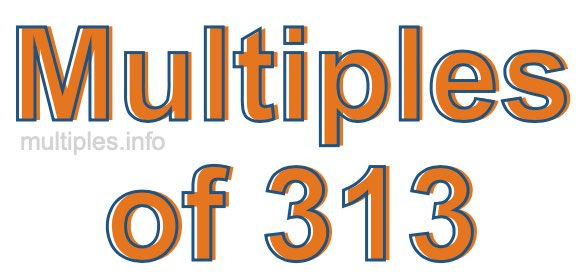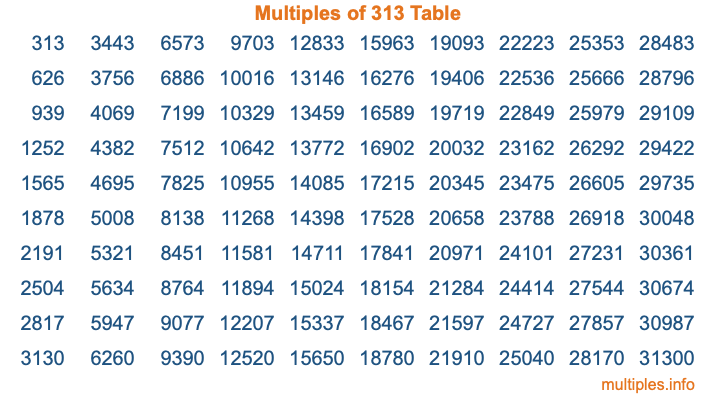Multiples of 313Welcome to the Multiples of 313 page. Here we will first teach you everything you will ever need to know about the multiples of 313, and then give you a study guide summary of everything we taught you to make sure you remember it all. Use this page to look up facts and learn information about the multiples of 313. This page will make you a multiples of three hundred thirteen expert!

Definition of Multiples of 313
Multiples of 313 are all the numbers that when divided by 313 equal an integer. Each of the multiples of 313 are called a multiple. A multiple of 313 is created by multiplying 313 by an integer.

Therefore, to create a list of multiples of 313, you start with 1 multiplied by 313, then 2 multiplied by 313, then 3 multiplied by 313, and so on for as long as you want. Thus, the list of the first five multiples of 313 is 313, 626, 939, 1252, and 1565. To see a larger list of multiples of 313, see the printable image of Multiples of 313 further down on this page. We also have a category where you can choose any nth multiple of 313.

Multiples of 313 Checker
The Multiples of 313 Checker below checks to see if any number of your choice is a multiple of 313. In other words, it checks to see if there is any number (integer) that when multiplied by 313 will equal your number. To do that, we divide your number by 313. If the the quotient is an integer, then your number is a multiple of 313.

Is  a multiple of 313?

Least Common Multiple of 313 and ...
A Least Common Multiple (LCM) is the lowest multiple that two or more numbers have in common. This is also called the smallest common multiple or lowest common multiple and is useful to know when you are adding our subtracting fractions. Enter one or more numbers below (313 is already entered) to find the LCM.

Check out our LCM Calculator if you need more details about the Least Common Multiple or if you need the LCM for different numbers for adding and subtraction fractions.

nth Multiple of 313
As we stated above, 313 is the first multiple of 313, 626 is the second multiple of 313, 939 is the third multiple of 313, and so on. Enter a number below to find the nth multiple of 313.

th multiple of 313

Multiples of 313 vs Factors of 313
313 is a multiple of 313 and a factor of 313, but that is where the similarities end. All postive multiples of 313 are 313 or greater than 313. All positive factors of 313 are 313 or less than 313.

Below is the beginning list of multiples of 313 and the factors of 313 so you can compare:

Multiples of 313: 313, 626, 939, 1252, 1565, etc.

Factors of 313: 1, 313

As you can see, the multiples of 313 are all the numbers that you can divide by 313 to get a whole number. The factors of 313, on the other hand, are all the whole numbers that you can multiply by another whole number to get 313.

It's also interesting to note that if a number (x) is a factor of 313, then 313 will also be a multiple of that number (x).

Multiples of 313 vs Divisors of 313
The divisors of 313 are all the integers that 313 can be divided by evenly. Below is a list of the divisors of 313.

Divisors of 313: 1, 313

The interesting thing to note here is that if you take any multiple of 313 and divide it by a divisor of 313, you will see that the quotient is an integer.

Multiples of 313 Table
Below is an image of the first 100 multiples of 313 in a table. The table is in chronological order, column by column. The first column has the first ten multiples of 313, the second column has the next ten multiples of 313, and so on.The Multiples of 313 Table is also referred to as the 313 Times Table or Times Table of 313. You are welcome to print out our table for your studies.

Negative Multiples of 313
Although not often discussed or needed in math, it is worth mentioning that you can make a list of negative multiples of 313 by multiplying 313 by -1, then by -2, then by -3, and so on, to get the following list of negative multiples of 313:

-313, -626, -939, -1252, -1565, etc.

Multiples of 313 Summary
Below is a summary of important Multiples of 313 facts that we have discussed on this page. To retain the knowledge on this page, we recommend that you read through the summary and explain to yourself or a study partner why they hold true.

There are an infinite number of multiples of 313.

A multiple of 313 divided by 313 will equal a whole number.

313 divided by a factor of 313 equals a divisor of 313.

The nth multiple of 313 is n times 313.

The largest factor of 313 is equal to the first positive multiple of 313.

313 is a multiple of every factor of 313.

313 is a multiple of 313.

A multiple of 313 divided by a divisor of 313 equals an integer.

313 divided by a divisor of 313 equals a factor of 313.

Any integer times 313 will equal a multiple of 313.

Multiples of a Number
Here you can get the multiples of another number, all with the same attention to detail as we did for multiples of 313 on this page.

Multiples of
Multiples of 314
Did you find our page about multiples of three hundred thirteen educational? Do you want more knowledge? Check out the multiples of the next number on our list!